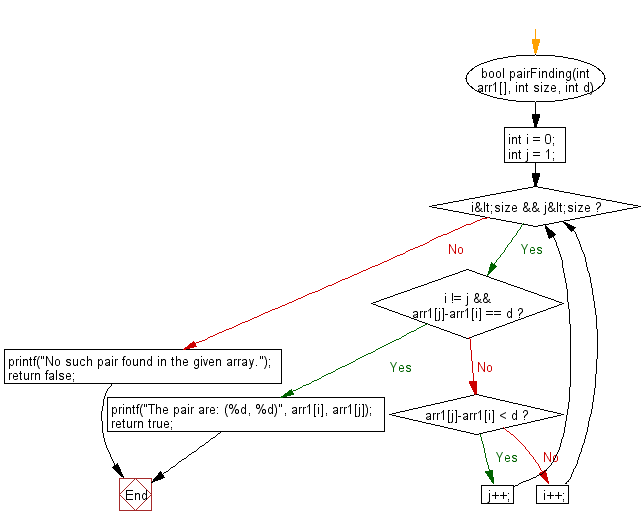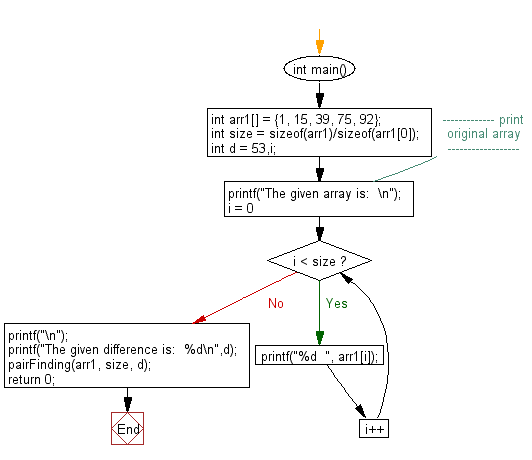﻿ C exercises: Find a pair with the given difference - w3resource# C Exercises: Find a pair with the given difference

## C Array: Exercise-83 with Solution

Write a program in C to find a pair with the given difference.

Sample Solution:

C Code:

``````#include <stdio.h>
# include <stdbool.h>

bool pairFinding(int arr1[], int size, int d)
{
int i = 0;
int j = 1;

while (i<size && j<size)
{
if (i != j && arr1[j]-arr1[i] == d)
{
printf("The pair are: (%d, %d)", arr1[i], arr1[j]);
return true;
}
else if (arr1[j]-arr1[i] < d)
j++;
else
i++;
}

printf("No such pair found in the given array.");
return false;
}

int main()
{
int arr1[] = {1, 15, 39, 75, 92};
int size = sizeof(arr1)/sizeof(arr1);
int d = 53,i;
//------------- print original array ------------------
printf("The given array is:  \n");
for(i = 0; i < size; i++)
{
printf("%d  ", arr1[i]);
}
printf("\n");
//------------------------------------------------------
printf("The given difference is:  %d\n",d);
pairFinding(arr1, size, d);
return 0;
}
```
```

Sample Output:

```The given array is:
1  15  39  75  92
The given difference is:  53
The pair are: (39, 92)
```

Pictorial Presentation:Flowchart 1:Flowchart 2:C Programming Code Editor:

Improve this sample solution and post your code through Disqus.

﻿